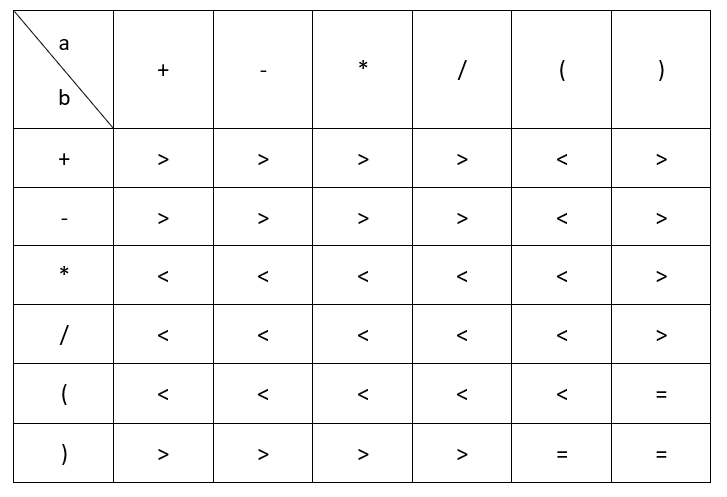a < b    a的优先级小于b的优先级

a = b    a的优先级等于b的优先级

a > b    a的优先级大于b的优先级```/**
* 算符集合用字符类型的数组表示
*/
char[] operator = {'+','-','*','/','(',')'};

/**
* 算符优先级矩阵用字符类型的二维数组表示
*/
char[][] matrix = {{'>','>','>','>','<','>'},
{'>','>','>','>','<','>'},
{'<','<','<','<','<','>'},
{'<','<','<','<','<','>'},
{'<','<','<','<','<','='},
{'>','>','>','>','=','='}
};```

```package com.milihua.algorithm.lineartable;
import java.util.Stack;
public class Evaluation {

/**
* 算符集合用字符类型的数组表示
*/
char[] operator = {'+','-','*','/','(',')'};

/**
* 算符优先级矩阵用字符类型的二维数组表示
*/
char[][]  matrix  = {{'>','>','>','>','<','>'},
{'>','>','>','>','<','>'},
{'<','<','<','<','<','>'},
{'<','<','<','<','<','>'},
{'<','<','<','<','<','='},
{'>','>','>','>','=','='}
};

/*
* 表达式求值
*/
public  float evaluate(String expression) {

char[] tokens = expression.toCharArray();
Stack<Float> stackOfNum = new Stack<Float>();
Stack<Character> stackOfOps = new Stack<Character>();
for (int i = 0; i < tokens.length; i++) {
if (tokens[i] == ' ')
continue;
if (tokens[i] >= '0' && tokens[i] <= '9') {
StringBuffer sbuf = new StringBuffer();
while (i < tokens.length && tokens[i] >= '0' && tokens[i] <= '9') {
sbuf.append(tokens[i++]);
}
i--; // 回退一位
stackOfNum.push(Float.parseFloat(sbuf.toString()));
}
if( isOperator(tokens[i]) )
{
if( stackOfOps.empty() )
{
stackOfOps.push(tokens[i]);
}
else
{
char ch = hasPrecedence(tokens[i], stackOfOps.peek());
if( ch == '<' )
{
stackOfOps.push(tokens[i]);
}
else if( ch == '>' )
{
if( tokens[i] == ')' )
{
while(true)
{
if( stackOfOps.empty() )
break;
char op = hasPrecedence(tokens[i], stackOfOps.peek());
if( op == '=' )
{
stackOfOps.pop();
break;
}
else
{
stackOfNum.push(caculate(stackOfOps.pop(), stackOfNum.pop(), stackOfNum.pop()));
}
}
}
else
{
stackOfNum.push(caculate(stackOfOps.pop(), stackOfNum.pop(), stackOfNum.pop()));
stackOfOps.push(tokens[i]);
}
}
}
}
}
while (!stackOfOps.empty())
{
stackOfNum.push(caculate(stackOfOps.pop(), stackOfNum.pop(), stackOfNum.pop()));
}
return stackOfNum.pop();

}

/**
* 判断读入的字符是否是运算符
*/
public boolean isOperator(char op)
{
for(int i = 0; i < operator.length; i++ )
{
if( op == operator[i] )
return true;
}
return false;
}

/**
* 获取运算符在数组中的索引
*/
public int getOperator(char op)
{
for(int i = 0; i < operator.length; i++ )
{
if( op == operator[i] )
return i;
}
return -1;
}

/**
* 判断op1和op2的优先级
*
*/
public  char  hasPrecedence(char op1, char op2) {

int suffixop1 = getOperator(op1);
int suffixop2 = getOperator(op2);
if( suffixop1 == -1 || suffixop2 == -1 )
return 0;
return matrix[suffixop1][suffixop2];
}

/**
* 执行运算
*
*/
public  float caculate(char op, float b, float a) {
switch (op) {
case '+':
return a + b;
case '-':
return a - b;
case '*':
return a * b;
case '/':
if (b == 0) {
throw new UnsupportedOperationException("Cannot divide by zero");
}
return a / b;
}
return 0;

}

/**
* 输出栈
*
*/
public void display(Stack  s)
{
for( int i = 0; i < s.size(); i++ )
{
System.out.print(s.get(i) + " ");
}
System.out.println();
}
}```

evaluate函数是算法的核心，基本体现了前面给出的算法思想。其中对小括号做了特殊处理，当遇到算符')'时，需要对算符栈连续执行出栈操作，并执行相关运算，直至与栈顶运算符优先级相等或者栈已空。

hasPrecedence函数用于判断两个算符的优先级关系，算符的优先级矩阵由matrix给出，函数返回矩阵给出的优先级关系。

```package unittest;
import com.milihua.algorithm.lineartable.Evaluation;
import com.milihua.algorithm.lineartable.Expression;
public class EvluationTest {

public static void main(String[] args) {
// TODO Auto-generated method stub
Evaluation  evaluation = new Evaluation();
System.out.println(evaluation.evaluate("2*(3+5)"));
System.out.println(evaluation.evaluate("100 * 2 + 12"));
System.out.println(evaluation.evaluate("100 * ( 2 + 12 )"));
System.out.println(evaluation.evaluate("100 * ( 2 + 12 ) / 14"));
System.out.println(Expression.evaluate("5 * ( 6 + 12 ) * 5 - 12"));
System.out.println(Expression.evaluate("3+12*18-60*15+3*(3+2)"));
}

}```

• 备案号：鲁ICP备15001146号
• @1997-2018 潍坊米粒花网络技术有限公司版权所有